# How time stepping works

GeoAnalytics Tools have multiple tools that allow you to analyze your data using time. There are two ways to analyze using time in GeoAnalytics Tools analysis.

1. Using temporal relationships.
2. Using time steps. Time steps slice your input data for analysis into steps defined by three parameters (time step interval, time step repeat interval and time step reference). When you apply time steps, analysis is completed on each time step independent of data outside of the time step of interest.

The output of time steps result in a feature service with fields representing the start time and end time of the time step interval. Using the fields START_DATETIME and END_DATETIME you can visualize your results over time.

## Time step summary

Time steps are a way of examining and analyzing your data through specified time intervals. Using three parameters, time interval, time step repeat interval, and reference time, you can specify the time steps you are interested in analyzing.

• Time step interval—the duration of the step you are interested in analyzing
• Time step repeat interval—how frequently you measure the time step interval
• Reference time—where in time to align the time step interval and time step repeat to, with time steps continuing both forwards and backwards from the reference time

How these three parameters work together is explained below using examples.

## Examples

### Time step interval only

The most basic time step is only specified by a time step interval (for example, 1 hour). In this example, these steps would result in four time steps. The slicing is automatically aligned at January 1st, 1970, and steps are calculated from that point. Time steps that are empty do not have any features returned.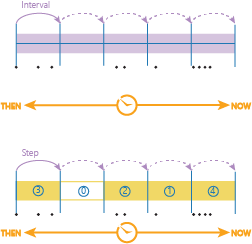### Time step interval and a reference time

If you wanted to specify a time for the time steps to begin at (instead of the default January 1, 1970), you can specify a reference time. A reference time can be a date (January 1, 2016) or a time and date (January 1, 2016 at 9:30 am). You cannot only apply a time (9:30 am). When applying a reference time, the time steps shift from the previous example, and now aligns with the reference time (new: dark blue, previous example, light blue).

This example has different results due to the reference time being used.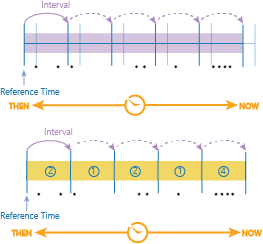### Time step interval and time step repeat interval

When using a time step interval without a repeat, the duration of your time step will always be equal to the time step. You can set a different time step repeat interval and time step interval so that analysis is completed with breaks between the time steps . For example, if you did a time step interval of 1 day, and a time step repeat interval of 1 hour, you would get the first hour of the day (that starts at the default reference time January 1, 1970).

In this example, there are for 4 time steps (yellow time steps with blue numbers).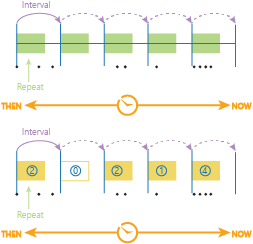### Time step interval, time step repeat, and reference time

Using all three parameters gives you the most control of your time steps. You can specify how often to analyze on time, how long, and what your steps are aligned to.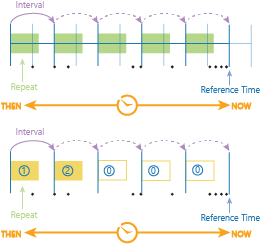Above is the old time alignment (light blue without arrows) that was determined by the default time start, and the new, user-defined alignment, determined by the reference time. The time steps and intervals have again shifted and that results are different than previous examples.

To give another example of what you can do; imagine you wanted to look at trends for data points that occurred every Monday. To calculate this you would set the following parameters: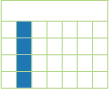• Time step interval: 1 week
• Time step repeat interval: 1 day
• Reference time: Monday January 1st, 2016.
##### Note:

Keep in mind that the reference time aligns both forward and backward. In this example, if your data wasn't in 2016, it would still properly align to the first of the month in any year after 1970.

## Time step and interval inputs

Time steps and intervals are input as string. The recognized time types are as follows:

• seconds
• minutes
• hours
• days
• weeks
• months
• years

## Time stepping results

When you analyze your data, and apply time stepping your output will be in the same geometry type (point, polyline, polygon) as if time wasn't enabled. Time stepping will always results in a time interval, which is represented by two fields in your output feature, the start_time and end_time. You can view your complete dataset without accounting for time, but you may have overlapping geometries. To correctly view your time stepped data, and how your results change over time use the time slider through ArcGIS Pro or the Map Viewer in your ArcGIS Enterprise portal. To learn more about using the time slider, see: